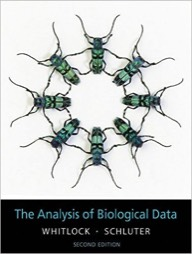## Introduction to Biostatistics

This course is an introduction to statistics and research design, including statistical inference, hypothesis testing, descriptive statistics and commonly used statistical tests. Emphasis is placed on the application of quantitative techniques in the biological sciences and solution methods via use of the computer.

Brought to you by:

# Description

This course is an introduction to statistics and research design, including statistical inference, hypothesis testing, descriptive statistics and commonly used statistical tests.  Emphasis is placed on the application of quantitative techniques in the biological sciences and solution methods via use of the computer.

# Detailed Syllabus

## Syllabus (Whitlock and Schluter):•    Chapter 1.  Statistics and samples (Random sampling, bias, types of data)
•    Chapter 2.  Displaying data
•    Chapter 3.  Describing data (Mean, variance, median, quartiles/quantiles)
•    Chapter 4.  Estimating with uncertainty (Standard error and confidence intervals)
•    Chapter 5.  Probability (Conditional probability and Bayes Theorem)
•    Chapter 6.  Hypothesis testing
•    Chapter 7.  Analyzing proportions (Binomial distribution)
•    Chapter 8.  Fitting probability models to frequency data (Chi-squared)
•    Chapter 9.  Contingency analysis: associations between categorical variables
•    Chapter 10.  The normal distribution (Central limit theorem)
•    Chapter 11.  Inference for a normal population (One sample t-test)
•    Chapter 12.  Comparing two means (Two sample t-test)
•    Chapter 13.  Handling violations of assumptions (Data transformations)
•    Chapter 15.  Comparing means of more than two groups (ANOVA)
•    Chapter 16.  Correlation between numerical variables
•    Chapter 17.  Regression (Linear, generalized linear and non-linear)
•    Chapter 18.  Multiple explanatory variables (General Linear Models)
•    Chapter 19.  Computer-intensive methods (Bootstrapping)
•    Chapter 20.  Likelihood (Maximum likelihood estimation)

## Syllabus (Ruxton and Colegrave):•    Chapter 1.  Why you should care about design (ethics; time; money; confounding factors)
•    Chapter 2.  Starting with a well-defined hypothesis (pilot studies; what is evidence?; field vs. laboratory; in vivo vs. in vitro)
•    Chapter 3.  Between-individual variation, replication, and sampling (replication and pseudoreplication; randomization; sampling errors)
•    Chapter 4.  Different experimental designs (controls; factorial design; blocking; split-plot designs)
•    Chapter 5.  Taking measurements (calibration; inaccuracy and imprecision; variability)
•    Chapter 6.  Final thoughts (treatment levels; subsampling; sampling techniques; covariates; human subjects)

## Syllabus (DataCamp):

This class is supported by DataCamp, the most intuitive learning platform for data science. Learn R, Python and SQL the way you learn best through a combination of short expert videos and hands-on-the-keyboard exercises. Take over 100+ courses by expert instructors on topics such as importing data, data visualization or machine learning and learn faster through immediate and personalised feedback on every exercise.